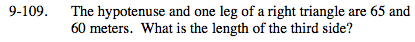### Home > MC2 > Chapter 9 > Lesson 9.3.3 > Problem9-109

9-109.

The hypotenuse and one leg of a right triangle are 65 and 60 meters. What is the length of the third side? Homework Help ✎Use the Pythagorean theorem.

(leg 1)2 + (leg 2)2 = (hypotenuse)2
Substitute 65 for the hypotenuse and 60 for leg 1.

(60)² + (leg 2)² = (65)²
Now simplify.

3600 + (leg 2)² = 4225
−3600 −3600
(leg 2)² = 625
Take the square root of each side.

$\sqrt{(\text{leg}\; 2)^{2}} = \sqrt{625}$

leg 2 = 25 meters